# Basic Excel Formulas

Time-saving ways to insert formulas into Excel

## Basic Excel Formulas Guide

Mastering the basic Excel formulas is critical for beginners to become highly proficient in financial analysis. Microsoft Excel is considered the industry standard piece of software in data analysis. Microsoft’s spreadsheet program also happens to be one of the most preferred software by investment bankers and financial analysts in data processing, financial modeling, and presentation. This guide will provide an overview and list of basic Excel functions.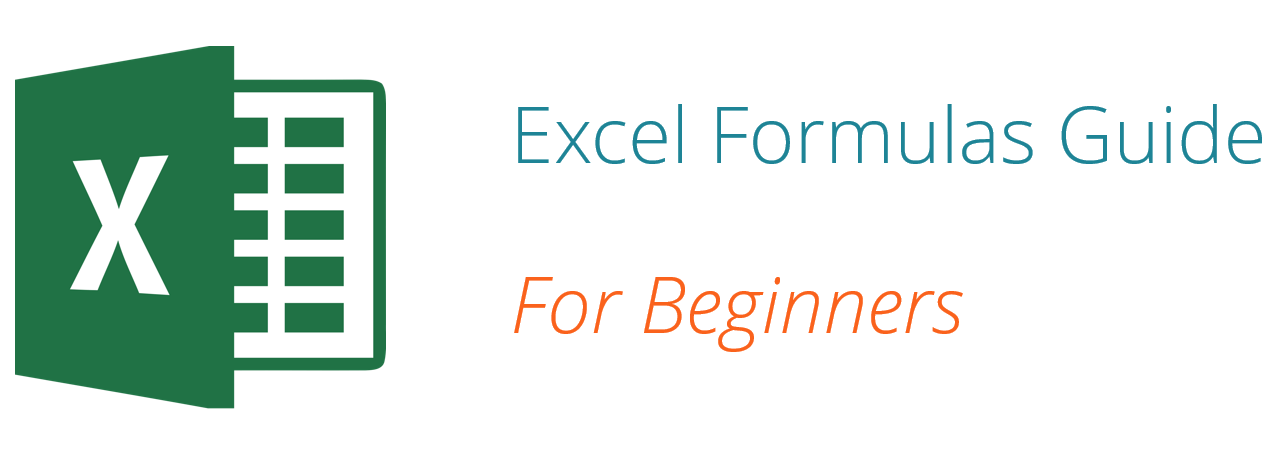Once you’ve mastered this list, move on to CFI’s advanced Excel formulas guide!

### Basic Terms in Excel

There are two basic ways to perform calculations in Excel: Formulas and Functions.

#### 1. Formulas

In Excel, a formula is an expression that operates on values in a range of cells or a cell. For example, =A1+A2+A3, which finds the sum of the range of values from cell A1 to cell A3.

#### 2. Functions

Functions are predefined formulas in Excel. They eliminate laborious manual entry of formulas while giving them human-friendly names. For example: =SUM(A1:A3). The function sums all the values from A1 to A3.

### Five Time-saving Ways to Insert Data into Excel

When analyzing data, there are five common ways of inserting basic Excel formulas. Each strategy comes with its own advantages. Therefore, before diving further into the main formulas, we’ll clarify those methods, so you can create your preferred workflow earlier on.

#### 1. Simple insertion: Typing a formula inside the cell

Typing a formula in a cell or the formula bar is the most straightforward method of inserting basic Excel formulas. The process usually starts by typing an equal sign, followed by the name of an Excel function.

Excel is quite intelligent in that when you start typing the name of the function, a pop-up function hint will show. It’s from this list you’ll select your preference. However, don’t press the Enter key. Instead, press the Tab key so that you can continue to insert other options. Otherwise, you may find yourself with an invalid name error, often as ‘#NAME?’. To fix it, just re-select the cell, and go to the formula bar to complete your function.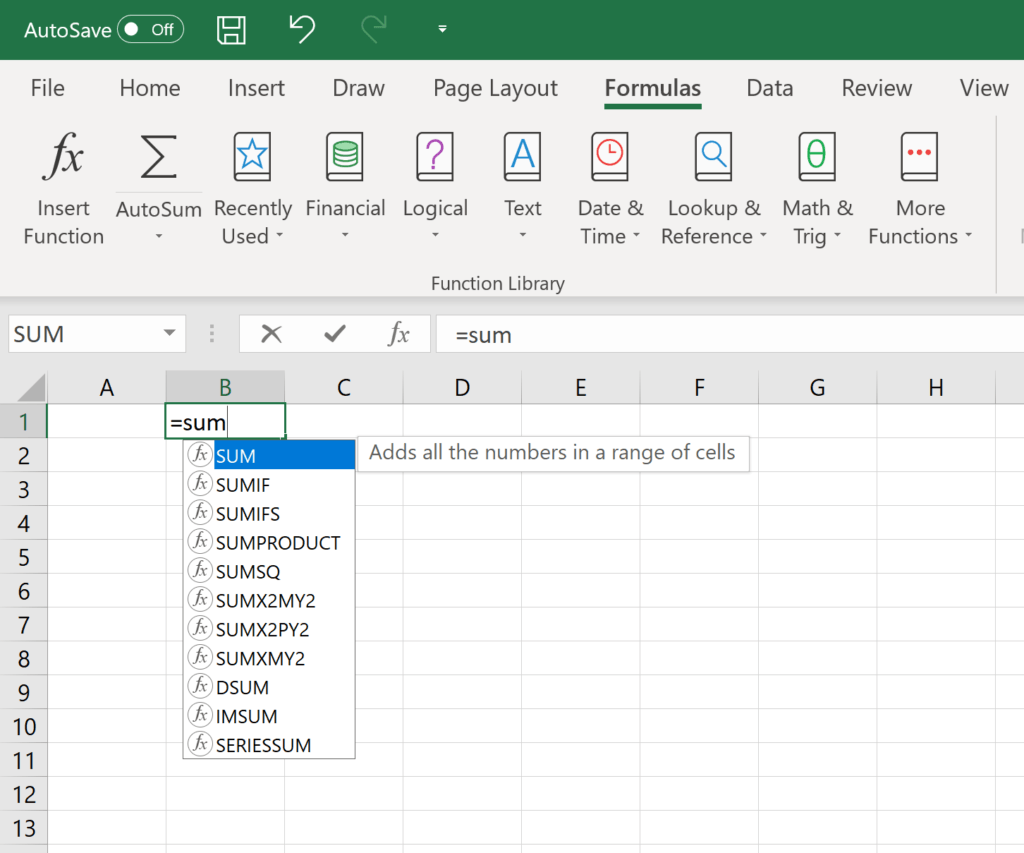Image: CFI’s Free Excel Crash Course.

#### 2. Using Insert Function Option from Formulas Tab

If you want full control of your functions insertion, using the Excel Insert Function dialogue box is all you ever need. To achieve this, go to the Formulas tab and select the first menu labeled Insert Function. The dialogue box will contain all the functions you need to complete your financial analysis.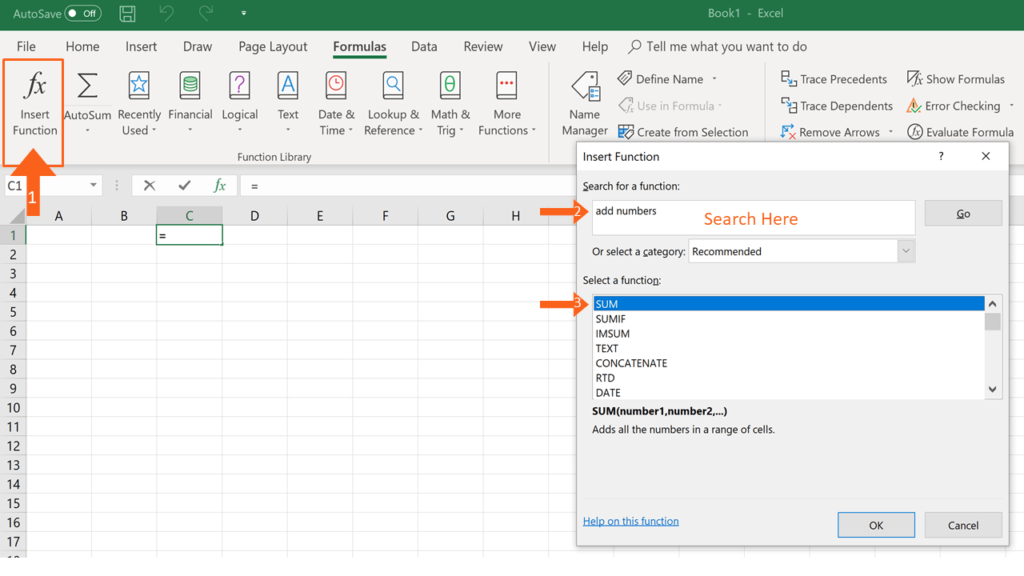#### 3. Selecting a Formula from One of the Groups in Formula Tab

This option is for those who want to delve into their favorite functions quickly. To find this menu, navigate to the Formulas tab and select your preferred group. Click to show a sub-menu filled with a list of functions. From there, you can select your preference. However, if you find your preferred group is not on the tab, click on the More Functions option – it’s probably just hidden there.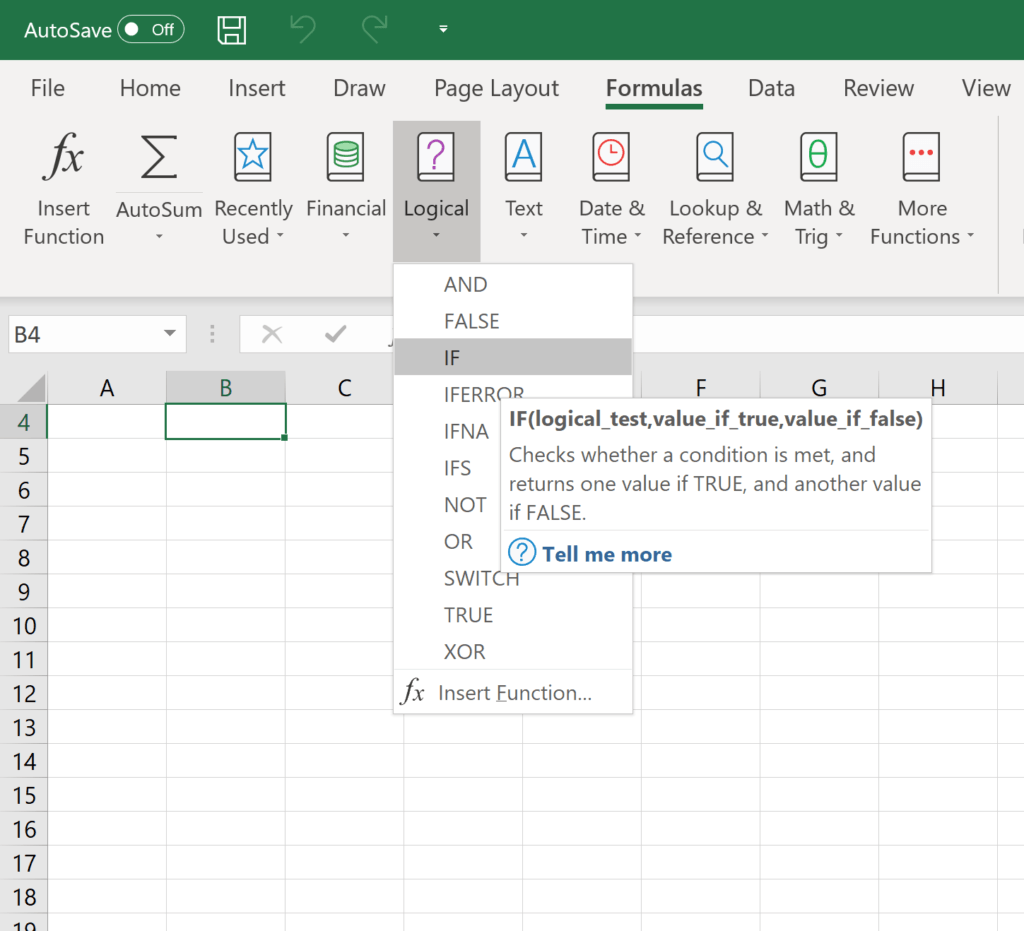Image: CFI’s Excel Courses.

#### 4. Using AutoSum Option

For quick and everyday tasks, the AutoSum function is your go-to option. So, navigate to the Home tab, in the far-right corner, and click the AutoSum option. Then click the caret to show other hidden formulas. This option is also available in the Formulas tab first option after the Insert Function option.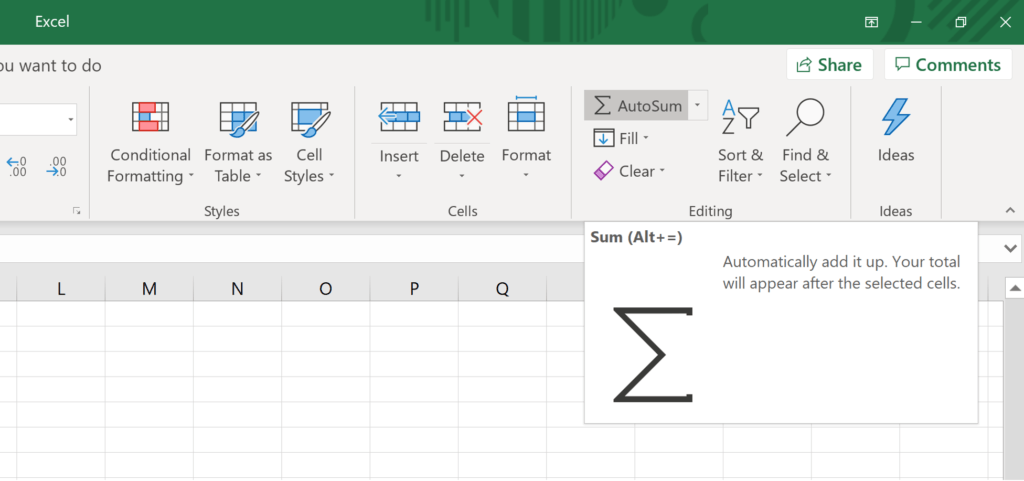#### 5. Quick Insert: Use Recently Used Tabs

If you find re-typing your most recent formula a monotonous task, then use the Recently Used menu. It’s on the Formulas tab, a third menu option just next to AutoSum.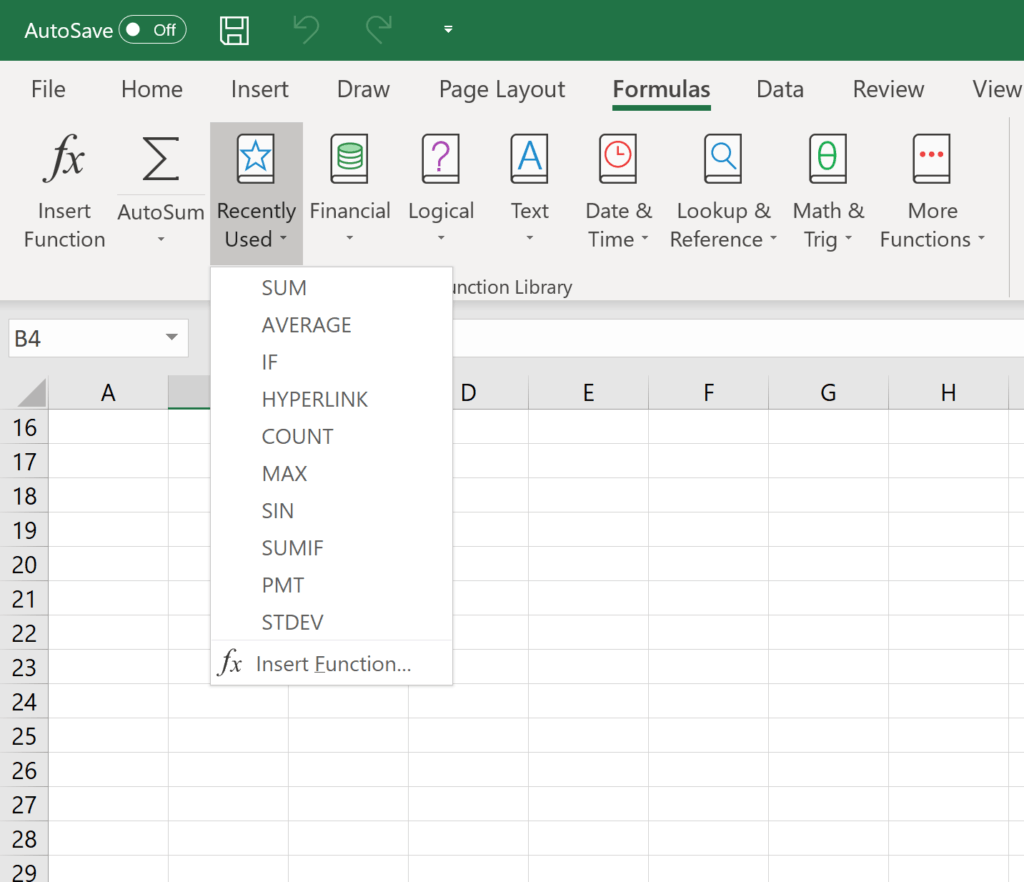### Free Excel Formulas YouTube Tutorial

Watch CFI’s FREE YouTube video tutorial to quickly learn the most important Excel formulas. By watching the video demonstration you’ll quickly learn the most important formulas and functions.

### Seven Basic Excel Formulas For Your Workflow

Since you’re now able to insert your preferred formulas and function correctly, let’s check some fundamental Excel functions to get you started.

#### 1. SUM

The SUM function is the first must-know formula in Excel. It usually aggregates values from a selection of columns or rows from your selected range.

=SUM(number1, [number2], …)

Example:

=SUM(B2:G2) – A simple selection that sums the values of a row.

=SUM(A2:A8) – A simple selection that sums the values of a column.

=SUM(A2:A7, A9, A12:A15) – A sophisticated collection that sums values from range A2 to A7, skips A8, adds A9, jumps A10 and A11, then finally adds from A12 to A15.

=SUM(A2:A8)/20 – Shows you can also turn your function into a formula.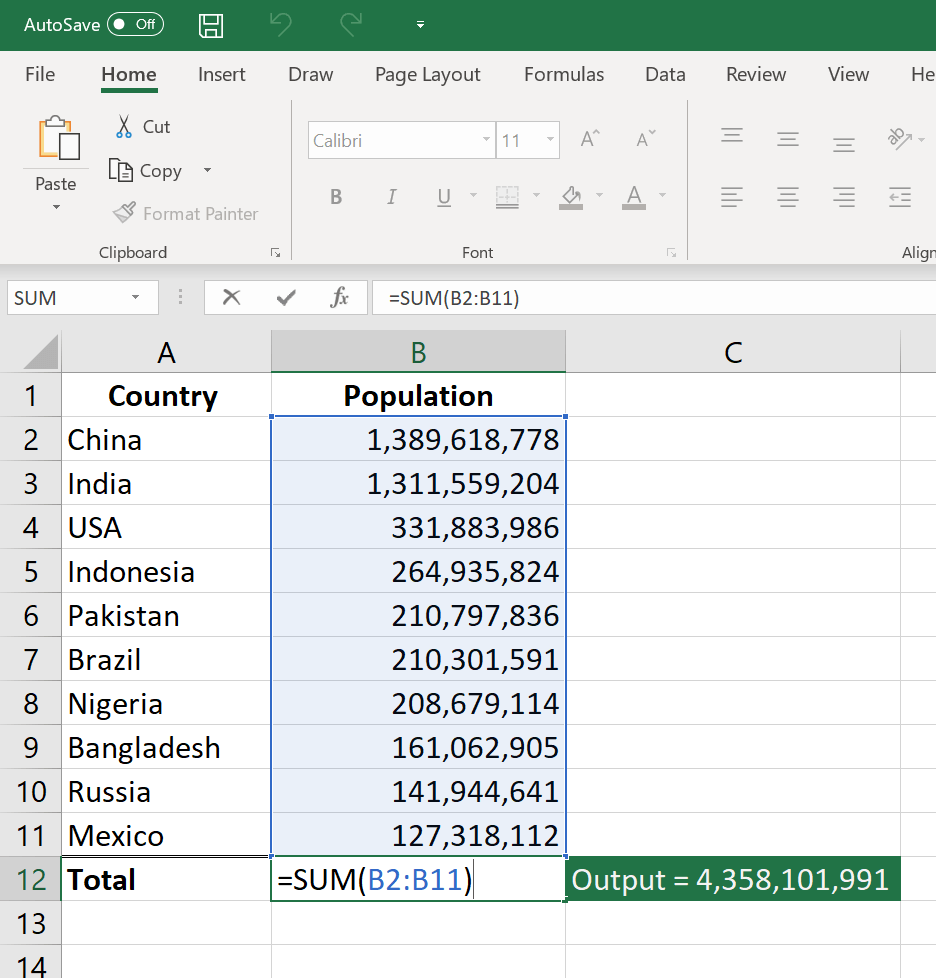Image: CFI’s Free Excel Crash Course.

#### 2. AVERAGE

The AVERAGE function should remind you of simple averages of data such as the average number of shareholders in a given shareholding pool.

=AVERAGE(number1, [number2], …)

Example:

=AVERAGE(B2:B11) – Shows a simple average, also similar to (SUM(B2:B11)/10)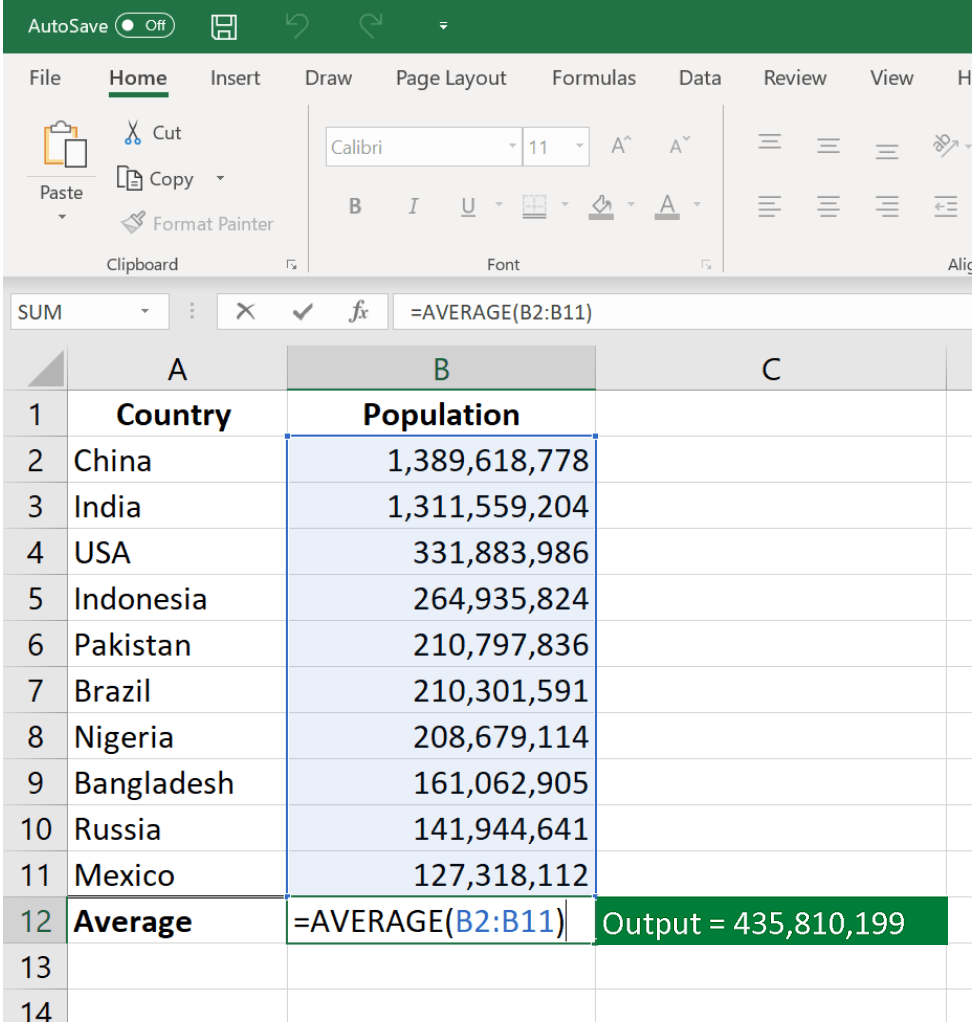#### 3. COUNT

The COUNT function counts all cells in a given range that contain only numeric values.

=COUNT(value1, [value2], …)

Example:

COUNT(A:A) – Counts all values that are numerical in A column. However, you must adjust the range inside the formula to count rows.

COUNT(A1:C1) – Now it can count rows.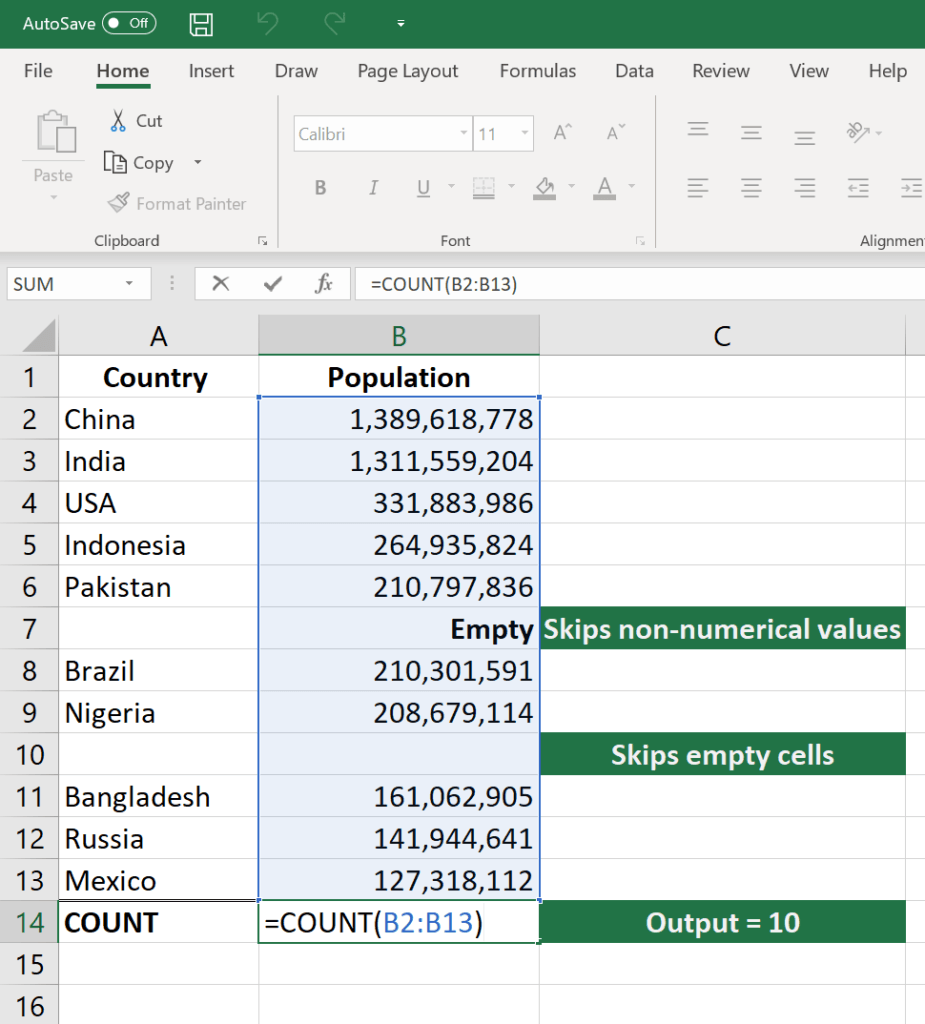Image: CFI’s Excel Courses.

#### 4. COUNTA

Like the COUNT function, COUNTA counts all cells in a given rage. However, it counts all cells regardless of type. That is, unlike COUNT that only counts numerics, it also counts dates, times, strings, logical values, errors, empty string, or text.

=COUNTA(value1, [value2], …)

Example:

COUNTA(C2:C13) – Counts rows 2 to 13 in column C regardless of type. However, like COUNT, you can’t use the same formula to count rows. You must make an adjustment to the selection inside the brackets – for example, COUNTA(C2:H2) will count columns C to H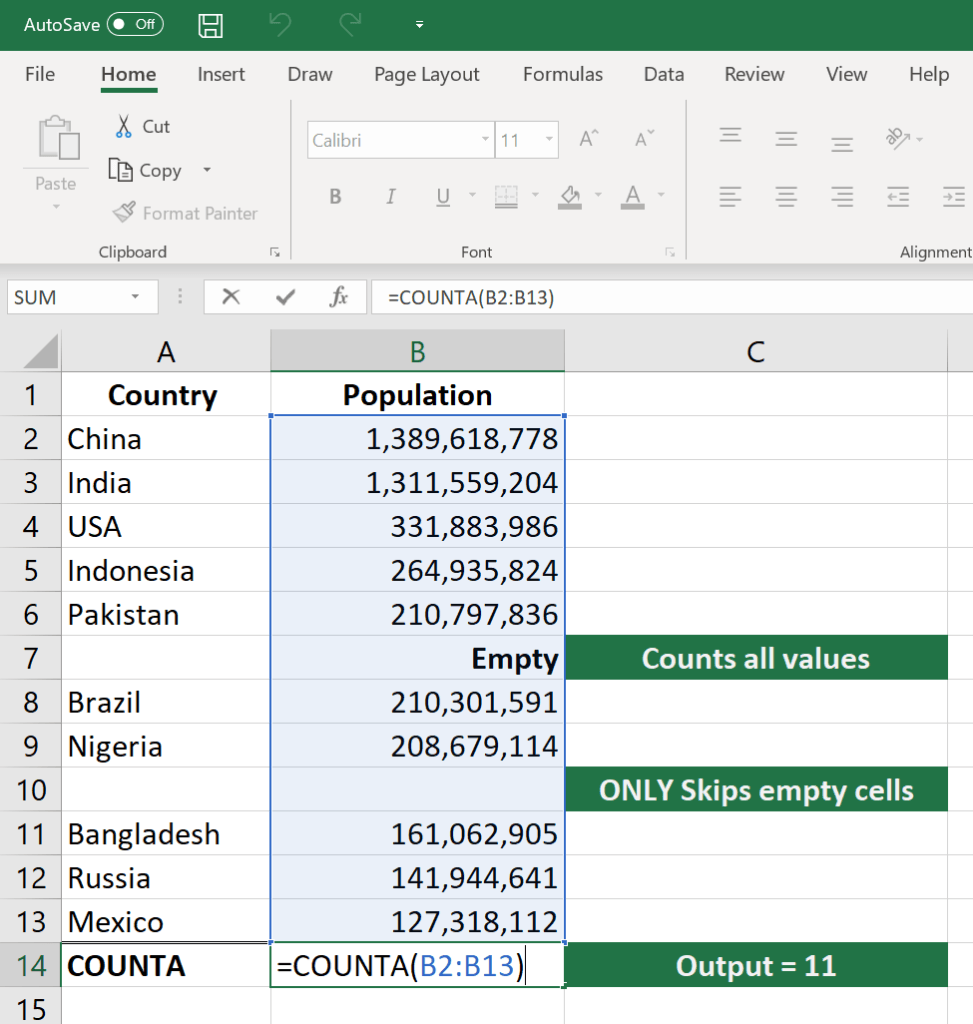#### 5. IF

The IF function is often used when you want to sort your data according to a given logic. The best part of the IF formula is that you can embed formulas and function in it.

=IF(logical_test, [value_if_true], [value_if_false])

Example:

=IF(C2<D3, ‘TRUE,’ ‘FALSE’) – Checks if the value at C3 is less than the value at D3. If the logic is true, let the cell value be TRUE, else, FALSE

=IF(SUM(C1:C10) > SUM(D1:D10), SUM(C1:C10), SUM(D1:D10)) – An example of a complex IF logic. First, it sums C1 to C10 and D1 to D10, then it compares the sum. If the sum of C1 to C10 is greater than the sum of D1 to D10, then it makes the value of a cell equal to the sum of C1 to C10. Otherwise, it makes it the SUM of C1 to C10.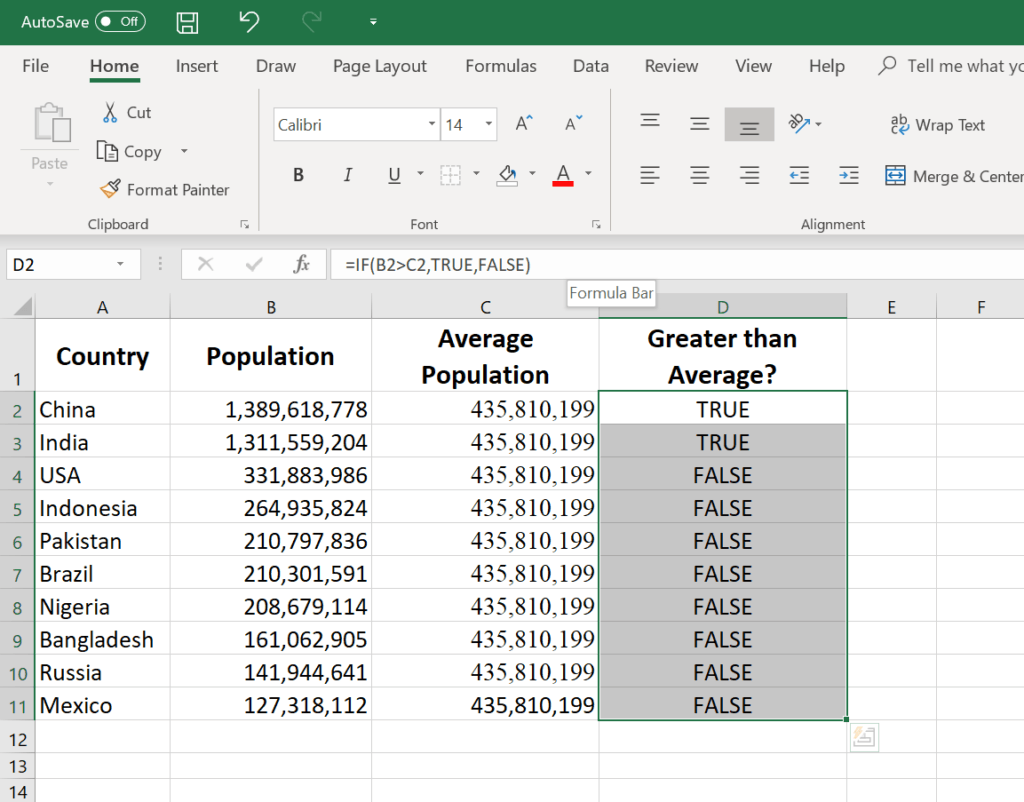#### 6. TRIM

The TRIM function makes sure your functions do not return errors due to unruly spaces. It ensures that all empty spaces are eliminated. Unlike other functions that can operate on a range of cells, TRIM only operates on a single cell. Therefore, it comes with the downside of adding duplicated data in your spreadsheet.

=TRIM(text)

Example:

TRIM(A2) – Removes empty spaces in the value in cell A2.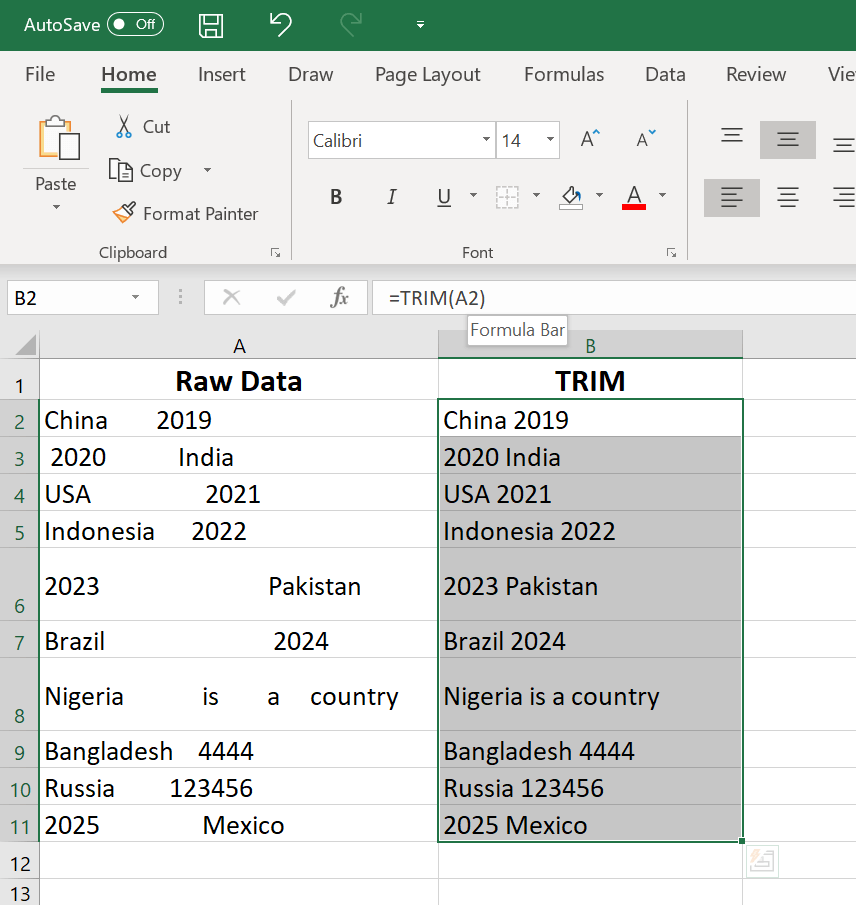Image: CFI’s Free Excel Crash Course.

#### 7. MAX & MIN

The MAX and MIN functions help in finding the maximum number and the minimum number in a range of values.

=MIN(number1, [number2], …)

Example:

=MIN(B2:C11) – Finds the minimum number between column B from B2 and column C from C2 to row 11 in both columns B and C.

=MAX(number1, [number2], …)

Example:

=MAX(B2:C11) – Similarly, it finds the maximum number between column B from B2 and column C from C2 to row 11 in both columns B and C.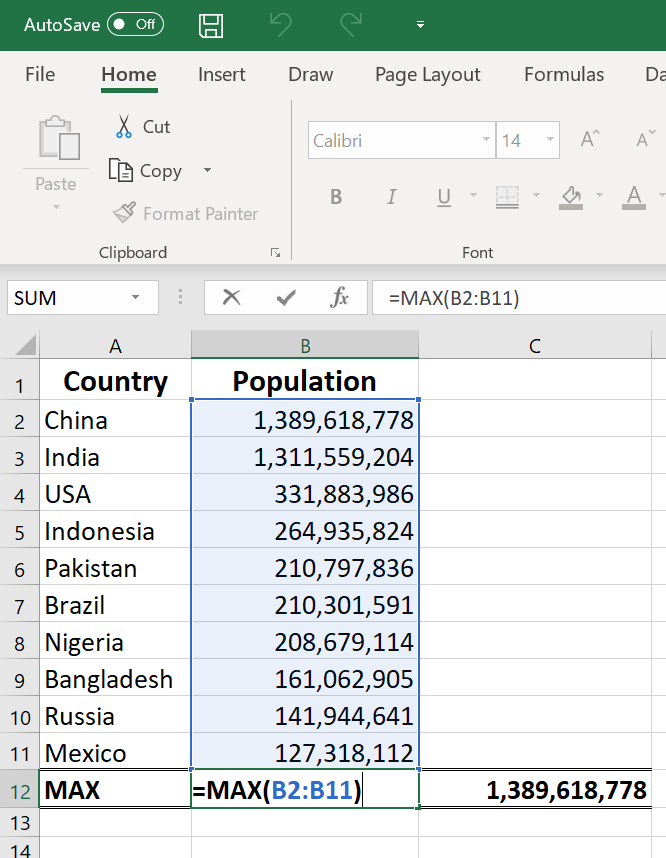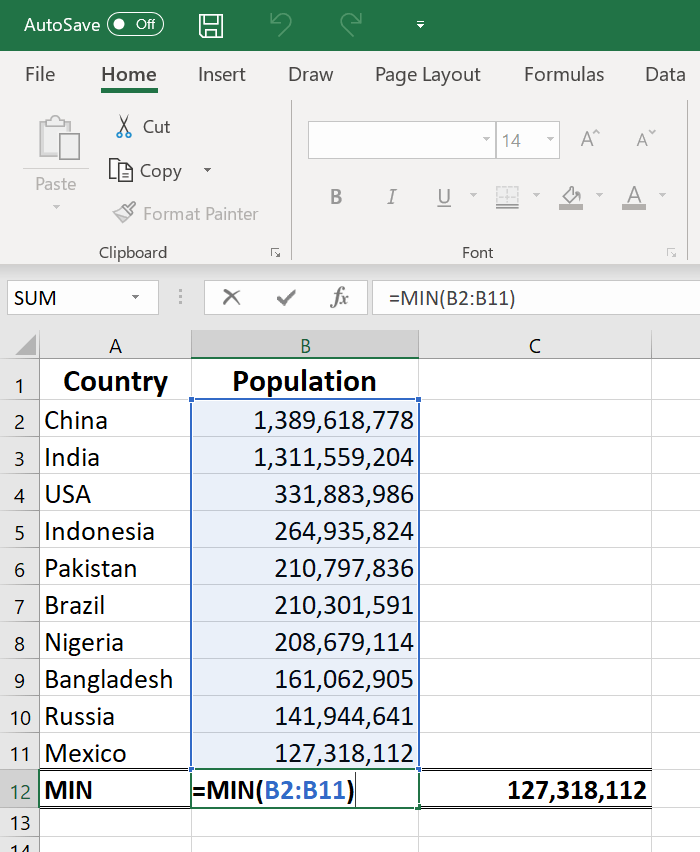### More Resources

Thank you for reading CFI’s guide to basic Excel formulas. To continue your development as a world-class financial analyst, these additional CFI resources will be helpful: